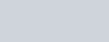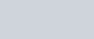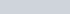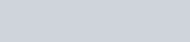ChemTalk

# Michaelis-Menten Equation

## Core Concepts

This article will explain how the Michaelis-Menten equation is used to understand enzyme kinetics. We’ll learn about the equation, how it can be visualized, and learn about its real world applications.

## Enzymes (Review)

To fully understand the Michaelis-Menten Equation, a significant knowledge on enzymes is required. Enzymes are a class of biological molecules that catalyze chemical reactions. They are essential to metabolic pathways and various physiological processes in all living things. Enzymes bind a specific substrate (molecule) at their active sites, forming an enzyme-substrate complex. Enzymes may have several active sites, or just one. The enzyme speeds up the conversion of the substrate into a product. Enzymes can do this over and over again, making them key to almost every metabolic process in the human body. To learn more about enzymes, read ChemTalk’s article!

## What is the Michaelis-Menten Equation?

The Michaelis-Menten Equation describes the relationship between the rate of an enzyme-catalyzed reaction and the concentration of the substrate. It was named after the scientists Leonor Michaelis and Maud Menten, who developed it in 1913. If you’ve read about kinetics, these words may sound familiar. That’s because enzyme-substrate reactions are chemical reactions! The equation can be written as follows:The equation can be written as above, where

• v is the initial velocity or rate of the enzymatic reaction.
• Vmax​ is the maximum velocity of the reaction, which occurs when all enzyme active sites are saturated with substrate.
• [S] is the concentration of the substrate.
• KM is the Michaelis constant, which is a measure of the enzyme’s affinity for its substrate. It is equal to the substrate concentration at which the reaction velocity is half of Vmax

## Substrate Affinity using the Michaelis-Menten Equation

While comparing various parts of the Michaelis-Menten equation, we can understand a lot about the enzyme-substrate complex.

Substrate affinity is the tendency of an enzyme to bind to a substrate. An enzyme with a high affinity easily binds the substrate, at a low concentration of substrate. When the variable KM is high, the enzyme has a low substrate affinity. In other words, the enzyme is less able to bind the substrate. Enzymes with a lower KM also tend to be better at converting substrates to products. The ability of an enzyme to convert the substrate to product is captured in the Vmax term in the Michaelis-Menten Equation. Enzymes that are more efficient catalysts will have higher (or faster) Vmax terms.

Let’s consider a few limiting cases of the Michaelis-Menten equation. When KM is so large it makes [S] negligible in the denominator, the equation simplifies toSituations like this require a higher [S], because the enzyme does not readily bind to the substrate. In this case, the enzyme has a low substrate affinity.

On the contrary, when KM is small, the equation simplifies toThis means the enzyme binds to the substrate easily, giving it a high substrate affinity. Then, the rate of the reaction only depends on the enzyme’s ability to catalyze the reaction.

## Lineweaver-Burk Plots

To clearly see the implications of the Michaelis-Menten equation, we can take the reciprocal to turn the equation into slope intercept form.Lineweaver-Burk graph this linearized form of the Michaelis-Menten equation. When we plot 1/vo agaisnt 1/[S], we can find Km and Vmax by looking at the slope and y intercept, respectively.

Lineweaver-Burk plots are a good way for scientists to visualize the parameters of an enzyme-substrate complex. Scientists can compare a number of different experimental conditions, inhibitors, or mutations in order to understand their impact on the enzyme kinetics.

## Michaelis-Menten equation using Steady State Approximation

The steady state approximation is an assumption, in the context of enzyme kinetics, that the rate of enzyme-substrate formation is equal to the rate of enzyme substrate disassociation.

The steady-state approximation is valid when the rate of formation and breakdown of the enzyme-substrate complex is much faster than the rate of other slower steps in the reaction, such as product formation or enzyme regeneration. Without the steady state approximation, we would not be able to derive the Michaelis-Menten equation. To learn more, read ChemTalk’s article!